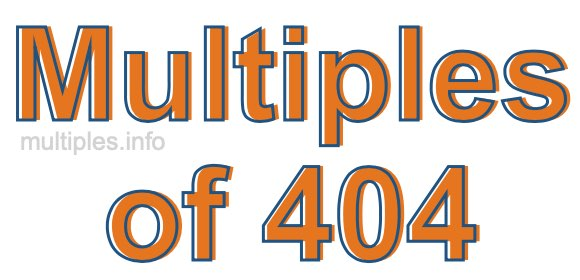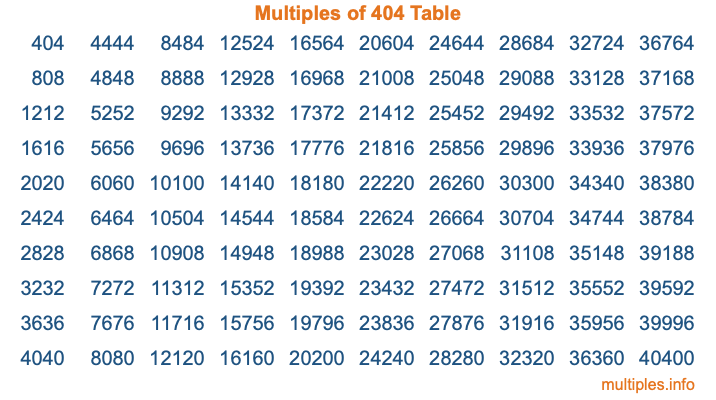Multiples of 404Welcome to the Multiples of 404 page. Here we will first teach you everything you will ever need to know about the multiples of 404, and then give you a study guide summary of everything we taught you to make sure you remember it all. Use this page to look up facts and learn information about the multiples of 404. This page will make you a multiples of four hundred four expert!

Definition of Multiples of 404
Multiples of 404 are all the numbers that when divided by 404 equal an integer. Each of the multiples of 404 are called a multiple. A multiple of 404 is created by multiplying 404 by an integer.

Therefore, to create a list of multiples of 404, you start with 1 multiplied by 404, then 2 multiplied by 404, then 3 multiplied by 404, and so on for as long as you want. Thus, the list of the first five multiples of 404 is 404, 808, 1212, 1616, and 2020. To see a larger list of multiples of 404, see the printable image of Multiples of 404 further down on this page. We also have a category where you can choose any nth multiple of 404.

Multiples of 404 Checker
The Multiples of 404 Checker below checks to see if any number of your choice is a multiple of 404. In other words, it checks to see if there is any number (integer) that when multiplied by 404 will equal your number. To do that, we divide your number by 404. If the the quotient is an integer, then your number is a multiple of 404.

Is  a multiple of 404?

Least Common Multiple of 404 and ...
A Least Common Multiple (LCM) is the lowest multiple that two or more numbers have in common. This is also called the smallest common multiple or lowest common multiple and is useful to know when you are adding our subtracting fractions. Enter one or more numbers below (404 is already entered) to find the LCM.

Check out our LCM Calculator if you need more details about the Least Common Multiple or if you need the LCM for different numbers for adding and subtraction fractions.

nth Multiple of 404
As we stated above, 404 is the first multiple of 404, 808 is the second multiple of 404, 1212 is the third multiple of 404, and so on. Enter a number below to find the nth multiple of 404.

th multiple of 404

Multiples of 404 vs Factors of 404
404 is a multiple of 404 and a factor of 404, but that is where the similarities end. All postive multiples of 404 are 404 or greater than 404. All positive factors of 404 are 404 or less than 404.

Below is the beginning list of multiples of 404 and the factors of 404 so you can compare:

Multiples of 404: 404, 808, 1212, 1616, 2020, etc.

Factors of 404: 1, 2, 4, 101, 202, 404

As you can see, the multiples of 404 are all the numbers that you can divide by 404 to get a whole number. The factors of 404, on the other hand, are all the whole numbers that you can multiply by another whole number to get 404.

It's also interesting to note that if a number (x) is a factor of 404, then 404 will also be a multiple of that number (x).

Multiples of 404 vs Divisors of 404
The divisors of 404 are all the integers that 404 can be divided by evenly. Below is a list of the divisors of 404.

Divisors of 404: 1, 2, 4, 101, 202, 404

The interesting thing to note here is that if you take any multiple of 404 and divide it by a divisor of 404, you will see that the quotient is an integer.

Multiples of 404 Table
Below is an image of the first 100 multiples of 404 in a table. The table is in chronological order, column by column. The first column has the first ten multiples of 404, the second column has the next ten multiples of 404, and so on.The Multiples of 404 Table is also referred to as the 404 Times Table or Times Table of 404. You are welcome to print out our table for your studies.

Negative Multiples of 404
Although not often discussed or needed in math, it is worth mentioning that you can make a list of negative multiples of 404 by multiplying 404 by -1, then by -2, then by -3, and so on, to get the following list of negative multiples of 404:

-404, -808, -1212, -1616, -2020, etc.

Multiples of 404 Summary
Below is a summary of important Multiples of 404 facts that we have discussed on this page. To retain the knowledge on this page, we recommend that you read through the summary and explain to yourself or a study partner why they hold true.

There are an infinite number of multiples of 404.

A multiple of 404 divided by 404 will equal a whole number.

404 divided by a factor of 404 equals a divisor of 404.

The nth multiple of 404 is n times 404.

The largest factor of 404 is equal to the first positive multiple of 404.

404 is a multiple of every factor of 404.

404 is a multiple of 404.

A multiple of 404 divided by a divisor of 404 equals an integer.

404 divided by a divisor of 404 equals a factor of 404.

Any integer times 404 will equal a multiple of 404.

Multiples of a Number
Here you can get the multiples of another number, all with the same attention to detail as we did for multiples of 404 on this page.

Multiples of
Multiples of 405
Did you find our page about multiples of four hundred four educational? Do you want more knowledge? Check out the multiples of the next number on our list!